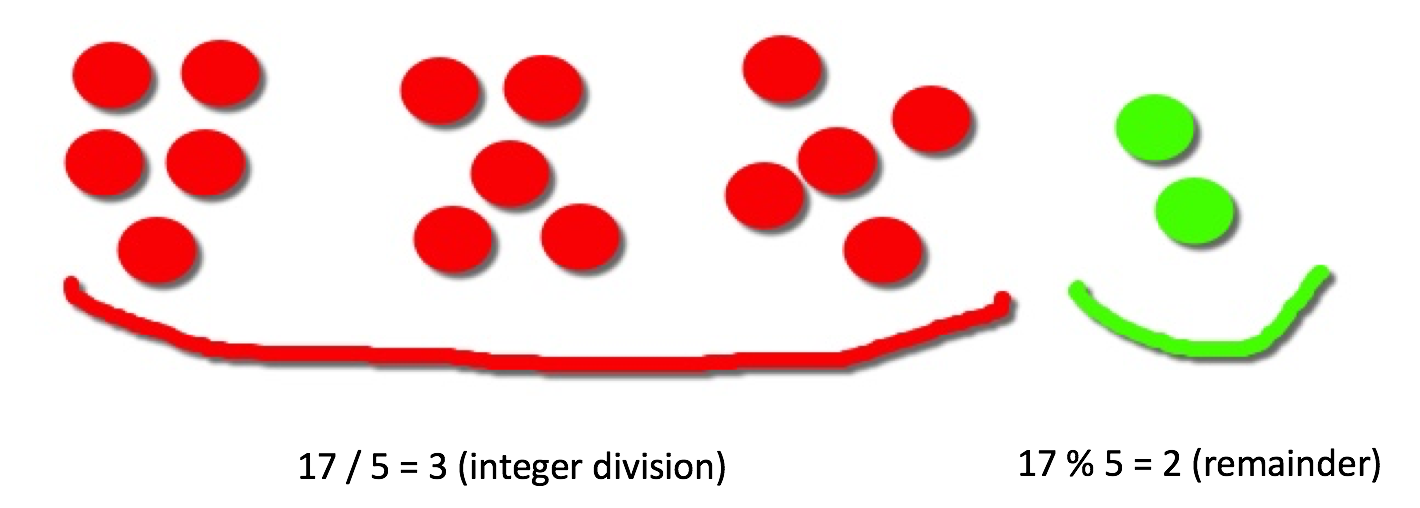In the first post of this series we start with a simple concept: the integer division and modulo operator (also called remainder operation). To demonstrate these concepts, we will use a classic whiteboard exercise, the Fizz Buzz. This is possibly the most widely used coding exercise in telephone and shared screen interviews.

The concept of integer division and modulo is very simple and can be better understood in the following graphical representation of 17/5:Let’s apply this concept to Fizz Buzz:

``````The Fizz Buzz

Write a method that returns 'Fizz' for multiples of three and 'Buzz' for the multiples of five.
For numbers which are multiples of both three and five return 'FizzBuzz'.
For numbers that are neither, return the input number.
``````
``````public String fizzBuzz(Integer i) {
String result = "";
return result;
}
``````

``````if (i % 3 == 0) {
result += "Fizz";
}
``````

The next step is to add Buzz for the multiples of five:

``````if (i % 5 == 0) {
result += "Buzz";
}
``````

Last step return the string representation of the number when the number is neither multiple of three or five.

``````if (result.equals("")) {
result = i.toString();
}
``````

All pieces assembled together we have:

``````public String fizzBuzz(Integer i) {
String result = "";
if (i % 3 == 0) {
result += "Fizz";
}
if (i % 5 == 0) {
result += "Buzz";
}
if (result.equals("")) {
result = i.toString();
}
return result;
}
``````

Feel free to play around with this exercise here.

You can find other exercises that use module operator and integer division here and here.